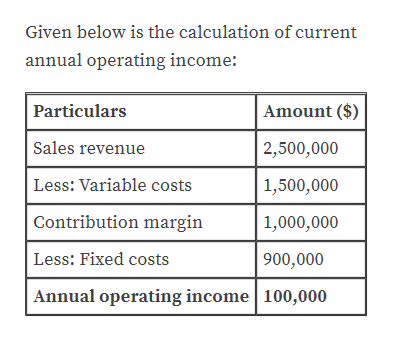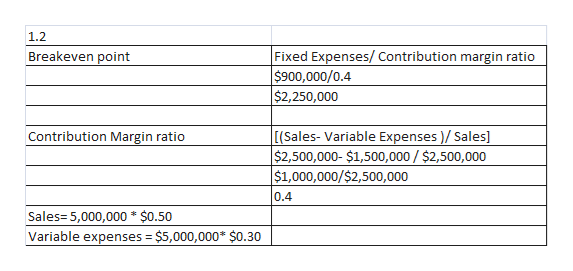# CVP exercises. The Doral Company manufactures and sells pens. Currently, 5,000,000 units are sold per year at \$0.50 per unit. Fixed costs are \$900,000 per year. Variable costs are \$0.30 per unit. Consider each case separately:What is the current annual operating income?What is the present breakeven point in revenues? Compute the new operating income for each of the following changes:A \$0.04 per unit increase in variable costsA 10% increase in fixed costs and a 10% increase in units soldA 20% decrease in fixed costs, a 20% decrease in selling price, a 10% decrease in variable cost per unit, and a 40% increase in units sold Compute the new breakeven point in units for each of the following changes:A 10% increase in fixed costs <b>6. </b>A 10% increase in selling price and a \$20,000 increase in fixed costs

Question
152 views

CVP exercises. The Doral Company manufactures and sells pens. Currently, 5,000,000 units are sold per year at \$0.50 per unit. Fixed costs are \$900,000 per year. Variable costs are \$0.30 per unit. Consider each case separately:

1. What is the current annual operating income?
2. What is the present breakeven point in revenues? Compute the new operating income for each of the following changes:
1. A \$0.04 per unit increase in variable costs
2. A 10% increase in fixed costs and a 10% increase in units sold
3. A 20% decrease in fixed costs, a 20% decrease in selling price, a 10% decrease in variable cost per unit, and a 40% increase in units sold Compute the new breakeven point in units for each of the following changes:
4. A 10% increase in fixed costs <b>6. </b>A 10% increase in selling price and a \$20,000 increase in fixed costs
check_circle

Step 1

“Hey, since there are multiple questions posted, we will answer three question. If you want any specific question to be answered then please submit that question only or specify the question number in your message.”

Step 2

1.1

Working note:

Sales revenue is calculated by multiplying 5,000,000 units * \$0.50

Variable expenses is calculated by multiplying 5,000,000 units * 0.30

Contribution margin of \$1,000,000 is calculated by subtracting \$2,500,000-\$1,500,000help_outlineImage TranscriptioncloseGiven below is the calculation of current annual operating income: Amount (\$) Particulars Sales revenue 2,500,000 Less: Variable costs |1,500,000 Contribution margin 1,000,000 Less: Fixed costs 900,000 Annual operating income 100,000 fullscreen
Step 3

1.1

...help_outlineImage Transcriptionclose1.2 Fixed Expenses/ Contribution margin ratio \$900,000/0.4 \$2,250,000 Breakeven point [(Sales- Variable Expenses )/ Sales] \$2,500,000- \$1,500,000 / \$2,500,000 \$1,000,000/\$2,500,000 Contribution Margin ratio 0.4 Sales= 5,000,000 * \$0.50 Variable expenses = \$5,000,000* \$0.30 %3D fullscreen

### Want to see the full answer?

See Solution

#### Want to see this answer and more?

Solutions are written by subject experts who are available 24/7. Questions are typically answered within 1 hour.*

See Solution
*Response times may vary by subject and question.
Tagged in